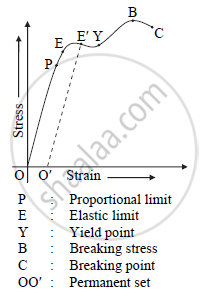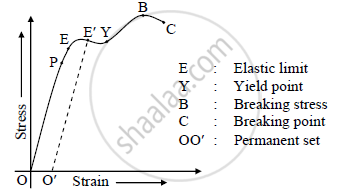Share

# Discuss the Behaviour of Wire Under Increasing Load. - Physics

#### Questions

Discuss the behaviour of wire under increasing load.

Explain the behaviour of metal wire under increasing load

#### Solution 1

Stress v/s strain graph:-The behaviour of wire under increasing load can be explained with the help of stress v/s strain graph.

a. Proportional limit: The initial portion OP of the graph is a straight line indicating stress is directly proportional to strain. Thus Hooke’s law is completely obeyed in the region OP. Point P is called point of proportional limit.

b. Elastic limit: Beyond the point P, the stress-strain variation is not a straight line as indicated by the part PE of the graph.

If the wire is unloaded at point E, the graph between stress and strain follows the reverse path EPO, then the point E is called elastic limit. The portion between O and E is called elastic region.

c. Permanent set: If the load is increased so that stress becomes greater than that corresponding to the point E, the graph is no longer a straight line and the wire does not obey Hooke's law. If the wire is strained upto E beyond point E and then the load is removed, the wire does not regain its original length and there is a permanent increase in length. A small strain corresponding to OO' is set up permanently in the wire, called permanent set. However, the wire is still elastic and if loaded again, gives a linear relation shown by the dotted line O'E'.

d. Yield point: As the stress is increased beyond the elastic limit the graph is a curve and reaches a point Y where the tangent to the curve is parallel to the strain axis. This shows that for the stress corresponding to point Y the strain increases even without any increase in the stress. This is known
as plastic flow.

Point Y on the curve is called yield point.

The value of stress corresponding to yield point is called yield stress.

e. Breaking stress: When the wire begins to flow, its cross-section decreases uniformly and hence, the stress increases steadily. Later a neck or constriction begins to form at a weak point. The maximum stress corresponding to the point B is breaking stress.

f. Breaking point: Once the neck is formed, the wire goes on stretching even if the load is reduced, until the breaking point C is reached when the wire breaks.

Elastic limit:-

The maximum stress to which an elastic body can be subjected without causing permanent deformation is called as elastic limit.

Yield point:-

The point on stress-strain curve at which the strain begins to increase without any increase in the stress is called yield point.

Breaking point:-

The point on stress-strain curve at which the wire breaks, is known as the breaking point.

#### Solution 2a) Elastic limit: The initial portion OE of the graph is a straight line, which indicates that upto the point E stress is directly proportional to strain. Hence, Hooke’s law is obeyed upto point E. In this region, wire is perfectly elastic and it completely regains its original length when the load is removed. Point E represents limit of proportionality between stress and strain.

b) Permanent set: If the load is increased so that stress becomes greater than that
corresponding to the point E, the graph is no longer a straight line and the wire does not obey Hooke's law.

If the wire is strained upto E′ beyond point E and then the load is removed, the wire does not regain its original length and there is a permanent increase in length. A small strain corresponding to OO' is set up permanently in the wire, called permanent set. However, the wire is still elastic and if loaded again, gives a linear relation shown by the dotted line O'E′.

c)Yield point: As the stress is increased beyond the elastic limit the graph is a
curve and reaches a point Y where the tangent to the curve is parallel to the strain
axis. This shows that for the stress corresponding to point Y the strain increases
even without any increase in the stress. This is known as plastic flow.
Point Y on the curve is called yield point.

The value of stress corresponding to yield point is called yield stress

e) Breaking point: Once the neck is formed, the wire goes on stretching even if the load is reduced, until the breaking point C is reached when the wire breaks.

Is there an error in this question or solution?